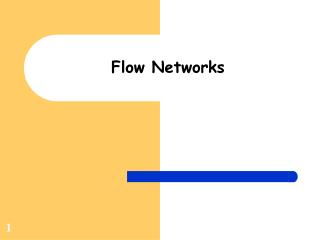DownloadDownload PresentationFlow Networks

# Flow Networks

Télécharger la présentation## Flow Networks

- - - - - - - - - - - - - - - - - - - - - - - - - - - E N D - - - - - - - - - - - - - - - - - - - - - - - - - - -
##### Presentation Transcript

1. Flow Networks

2. Flow Networks Directed Graph with edge capacities Capacities represent flow of materials Examples Water that can flow through a pipe Traffic that can flow between two intersections Electricity flowing through a wire Data transmitted over network connection

3. Maximum Flow Determine the max flow through the system Specify a source (s) and a sink (t) No edge carries more than its capacity For all vertices (other than s and t) flow in = flow out distributes flow over outgoing edges

4. Examples s s 3 2 7 19 4 1 a b a b 10 3 4 2 14 4 8 c d c d 6 2 3 4 20 t t

5. Flow Network (Formal Definition) • Flow network is a directed graph G = (V,E) • Each edge has non-negative capacity: • For each (u,v) in E, c(u,v) >= 0 • For each (u,v) not in E, c(u,v) = 0 • Contains a source s and sink t • s,t in V • Each vertex v in V is in some path from s to t

6. Flow in a Flow Network • Flow in G=(V,E) is a function f : V x V -> R • f satisfies properties • Capacity constraint f(u,v) <= c(u,v) for all u,v  V • Skew symmetry f(u,v) = -f(v,u) for all u,v  V • Flow conservation ∑f(u,v) = 0 for all u  V • Value of a flow: |f| = ∑f(s,v) • Maximum flow problem: maximize |f| v V v  V

7. Simple Algorithm Step 1: Choose any path from s to t Step 2: Determine maximum flow through path Step 3: Create flow and residual graphs

8. Keep track of augmentation paths Allow ‘reverse’ of previously chosen flow Ford-Fulkerson Method